Hyperbolic set

of a smooth dynamical system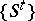A compact subsetof the phase manifold, completely consisting of trajectories, each of which has the following property: It has a neighbourhood such that all trajectories in that neighbourhood (including those which are not in) behave (with respect to the given trajectory) like the trajectories near a saddle. More exactly, a hyperbolic set in a smooth dynamical systemis a compact invariant subsetof the phase manifoldsuch that at each pointin the tangent space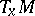tothere exist subspaces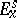andfor which the following two conditions are met: 1) the action of the differentials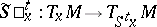of the mappings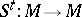aton the vectors,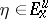satisfies the inequalities (cf. Differentiation of a mapping)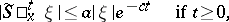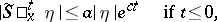with certain constantswhich are independent of. 2) Ifis a cascade (i.e. if the timeassumes integral values), thenifis a flow,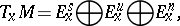where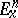is the one-dimensional subspace spanned by the vector of phase velocity (this involves the assumption that the latter does not vanish anywhere on). Moreover, for the sake of convenience in certain formulations, one calls equilibrium positions of flows for which the eigen values of the matrix of the linearized system lie outside the imaginary axis also hyperbolic (cf. Hyperbolic point).

The subspaceis called stable,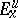is called unstable andis called neutral. The points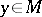for which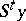andtend to each other as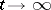form a smooth manifold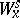tangent toat; it is called the stable manifold of the point. The union of thefor alllying on the same trajectory is called the stable manifold of this trajectory. The unstable manifolds of a point or a trajectory are defined in the same manner.

The classical example of a hyperbolic set of a flow is a periodic trajectory for which only one Floquet multiplier has modulus one. In certain systems the whole phase space is a hyperbolic set (cf.-system). Numerous examples of hyperbolic sets were discovered during the study of dynamical systems of classical origin — for example, in celestial mechanics . Hyperbolic sets as a class were introduced in 1965 by S. Smale , and have since played an important role in the theory of smooth dynamical systems, both as objects of studies and as a part in many examples .